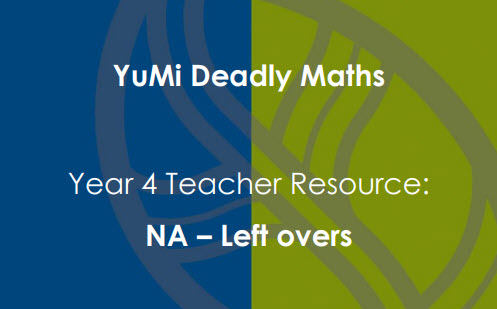# Left overs

Students investigate the relative position of numbers by modelling fractional numbers between whole numbers. They represent linear models of fractions along number lines and use these models to count and solve simple fraction addition problems.

Year level(s) Year 4
Audience Teacher
Purpose Teaching resource
Teaching strategies and pedagogical approaches Concrete Representational Abstract model, Culturally responsive pedagogies, Explicit teaching

## Curriculum alignment

Curriculum connections Numeracy
Strand and focus Number, Build understanding
Topics Fractions
AC: Mathematics (V9.0) content descriptions
AC9M4N03
Find equivalent representations of fractions using related denominators and make connections between fractions and decimal notation

AC9M4N04
Count by fractions including mixed numerals; locate and represent these fractions as numbers on number lines

Numeracy progression Interpreting fractions (P5)
Counting processes (P8)